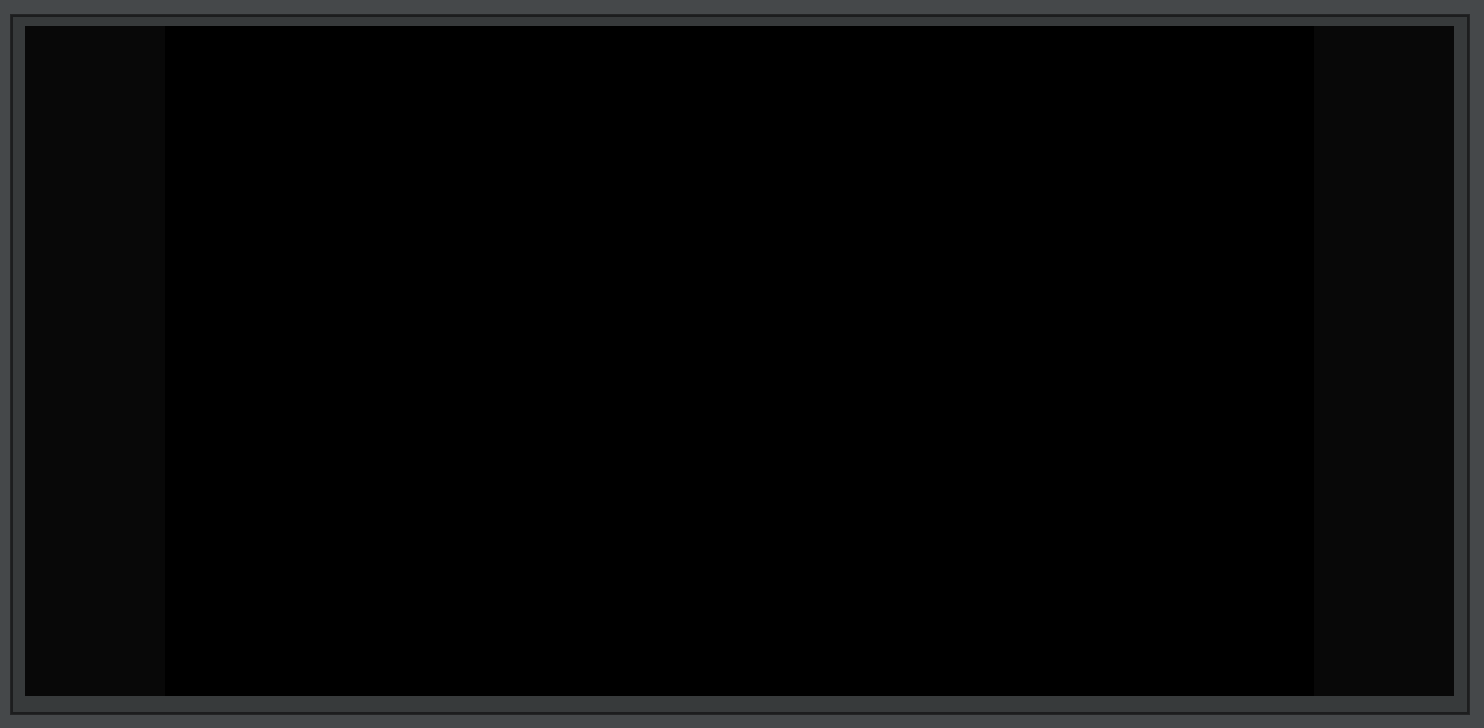# Full 3d Geometry

Much of Field and Field’s documentation is dedicated to making 2 and what you might call 2 and a half dimensional drawing. This is partly to provide an easy introduction to drawing with a computer, and partly due to the primacy of 2d drawing in general in art (canvases are flat). Obviously, everything is secretly 3D with `FLine`s being turned into 3d lines, points and triangles. While the FLine representation of shapes as filled flat areas is flexible, sometimes you’d like the real thing. Recent versions of Field expose an interface to this real thing that fits into the `Stage` / layer framework. This interface is more complex, more laborious and more powerful than `FLine`s alone.

## Getting started

First, make a `Stage` of your choosing and make some code to make a layer on that stage:What do we see with this? Nothing! Why? Two reasons: First, while we’ve added three vertices using `v(x,y,z)` to the triangle builder we got from the layer, we haven’t added any actual triangles. Vertices and the triangles that cover them are separate things (you can see this when you think about drawing a sphere — many triangles connect to each of the vertices). Let’s add a triangle:

`e(a,b,c)` adds a triangle over the vertices `a`, `b` and `c`. The numbering scheme here is that `0` refers to the most recent vertex vertex added (here `[0, 1.5, 0]`) and `1` refers the the next-most recent added and so on. This seems backwards at first, but it allows you to write code here that is independent of whatever else might be in the builder already (maybe some other code has already put 100 vertices in the builder before this code runs — you’d have to change to `.e(100,101,102)` if the numbering scheme wasn’t this way). If we asked for a `lineBuilder` then we’d be saying things like `.e(0,1)` to make a line segment out of two vertices rather than a triangle.

What do we see now?Still nothing. We haven’t set the color of the triangle. To do this, we need to add some extra information to the vertices:

This labels the first vertex as being a pale blue. Without any subsequent ‘aux’ commands, all other vertices end up with the same label.Finally, a triangle. But why does aux `1` set the color? If you are intrigued see the documentation on shaders. This is where `1` gets meaning. You can set other channels of information (up to 16) and have them appear in your shaders.

We can set aux information on every vertex:

Yields:Note how the color is being interpolated across the face of the triangle.

## Never doing that again!

Setting vertices by hand can get quite tedious. Two ways we avoid this: re-using existing code, and loading models from disk. Field comes with a rudimentary `Ply` file loader:

Here I’m using a broken yellow flower reconstruction curtesy of Tyler Araujo:Alternatively Field has a repository of standard shapes:

This draws a sphere (an approximation made, of course, out of, here, `15` slices of `10` circles of vertices). Sphere doesn’t set a color, so we have to do that up front (0.3, 0.6, 0.8, 1 is an opaque pale blue):If we want this to be ‘lit’ then, as you might expect, we need to edit the shader. After exposing this layers (triangle) shader to this box using `layer.bindTriangleShader(_)` edit the vertex shader to include this declaration near the top:

And then near the bottom, modulate that vertex color by a ‘lighting equation’:`new Spheres()` contains all kinds of other basic primitives. `Shapes.torus(1,20,20,0.5, someTriangles)` yields a torus: